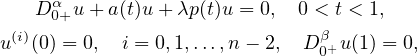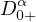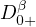Electron. J. Differential Equations, Vol. 2021 (2021), No. 62, pp. 1-14.

### Smallest eigenvalues for boundary value problems of two term fractional differential operators depending on fractional boundary conditions Paul W. Eloe, Jeffrey T. Neugebauer

Abstract:
Let n≥2 be an integer, and let n-1<α≤n. We consider eigenvalue problems for two point n-1 ,1 boundary value problemswhere 0≤ β ≤n-1 andanddenote standard Riemann-Liouville differential operators. We prove the existence of smallest positive eigenvalues and then obtain comparisons of these smallest eigenvalues as functions of both p and β.

Submitted October 14, 2020. Published July 7, 2021.
Math Subject Classifications: 26A33, 34A08, 34A40, 26D20
Key Words: Riemann-Liouville fractional differential equation; boundary value problem; principal eigenvalue; fractional boundary conditions
DOI: https://doi.org/10.58997/ejde.2021.62

Show me the PDF file (342 KB), TEX file for this article.Paul W. Eloe Department of Mathematics University of Dayton Dayton, OH 45469, USA email: peloe1@udayton.edu Jeffrey T. Neugebauer Department of Mathematics and Statistics Eastern Kentucky University Richmond, KY 40475, USA email: Jeffrey.Neugebauer@eku.edu